## User guide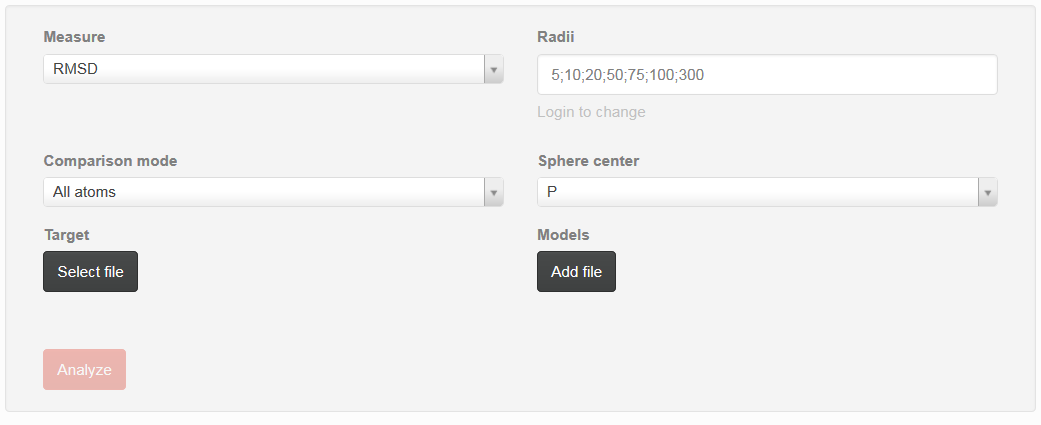Fig. 1. Screenshot of the User guide

User guide can be used to set up parameters that can be used during analysis (Fig. 1).

Measure
user can choose metrics that will be used for detailed analysis. One can choose between RMSD – root mean square deviation, Deformation Index, Adjusted RMSD (taking into consideration penalty if some atoms are missing), Interactions Network Fidelity All, Interactions Network Fidelity Watson-Crick, Interactions Network Fidelity non-WatsonCrick, Interactions Network Fidelity Stacking
correspond to vector of sphere radii that user wants to be analyzed. Each radius should be separated by semicolon
Comparison mode
user can choose between “all atoms” or “single atom” option:
• Single atom - from the whole molecule only correctness of selected type of atoms will be analyzed for reference structure and models
• All atoms - all atoms will be considered during evaluation of models quality against reference structure
Sphere center
user can choose atom that corresponds to the center of sphere that has been built on each nucleotide. One can choose between P, C1’, O5’, O3’
Target
selection of the reference structure
Models
selection of set of models that will be analyzed against reference structure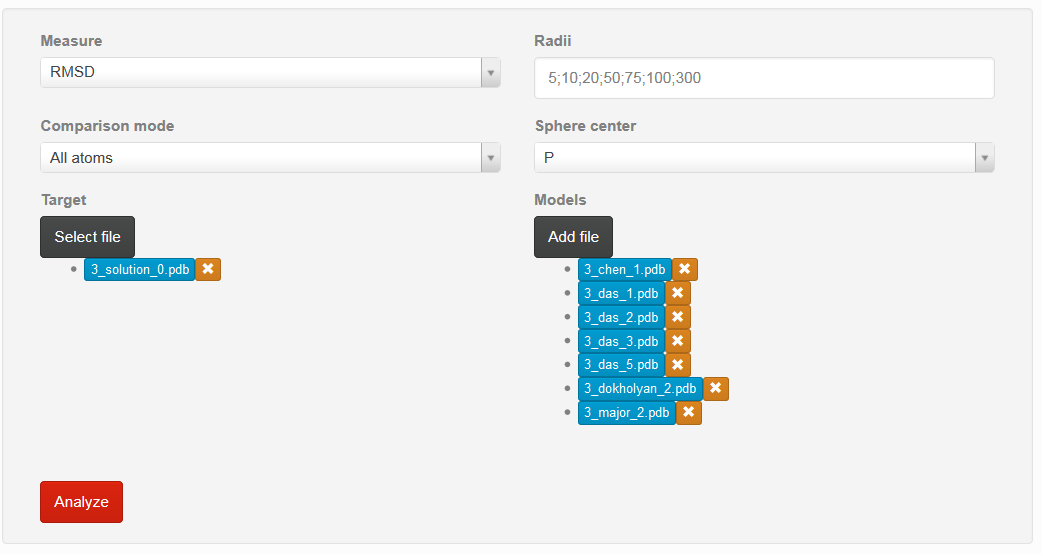Fig. 2. Screenshot of the User guide with chosen models and reference structure

If all parameters are correctly set, one can choose “Analyze” button to proceed with analysis (Fig. 2).

If user want to delete previously chosen structure, mark “x” situated on the right side of the particular model should be chosen.

By clicking on the name of model, user can obtain visualization of the particular structure.

#### Result of the analysis

Results section consists of three parts:

1. Global quality analysis
2. Linear plots
3. 2D and 3D graph

Each graph can be enlarged by simply clicking on it.

#### Ad a) Global quality analysis

That section is available if all metrics are calculated for the whole molecule. At the beginning one find only red bar, but after calculation one can see statistics for analyzed models (Fig. 3)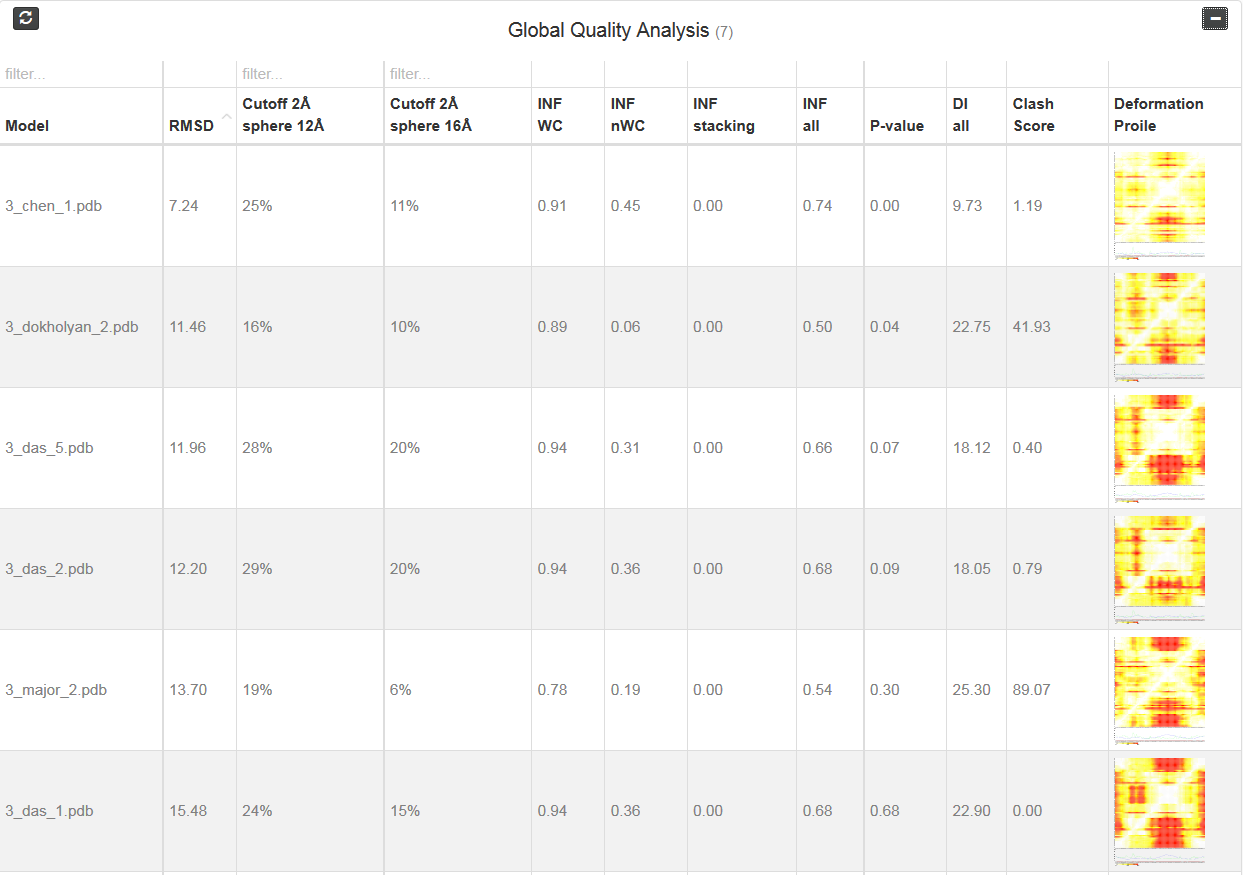Fig. 3. Global Quality analysis results

Each column correspond to one metrics. Additionally, one can see two “cutoff” metrics - the calculation is performed based on the fixed precision value (cutoff threshold) for each sphere radius, defined by the user in the spheres radii vector; as a result, the user receives information about the percentage of nucleotides predicted with the quality below specified cutoff (%). Last column corresponds to the picture of Deformation profile (Parisien et al., 2009). By double click user can enlarge the picture and view results of the analysis.

Parisien M, Cruz JA, Westhof E, Major F. 2009. New metrics for comparing and assessing discrepancies between RNA 3D structures and models. RNA 15(10):1875-1885.

That section consists of set of three type of plots: Averaged, cutoff and multimodel.

#### Averaged plot

An example of averaged plot is shown in Fig. 4. This is also a multiple model line plot, where each line describes exactly one structural model (different colors correspond to different models). The difference between Multi-model plot and averaged plot is that in the latter case the Y-axis represents values of metric calculated as the averaged sum of value of spheres built with fixed radius for all nucleotides of the analyzed RNA molecule. This plot visualizes how averaged values change with an increasing sphere radius. In general, this plot describes the changes of quality of prediction from the local structural neighborhood to the whole molecule point of view.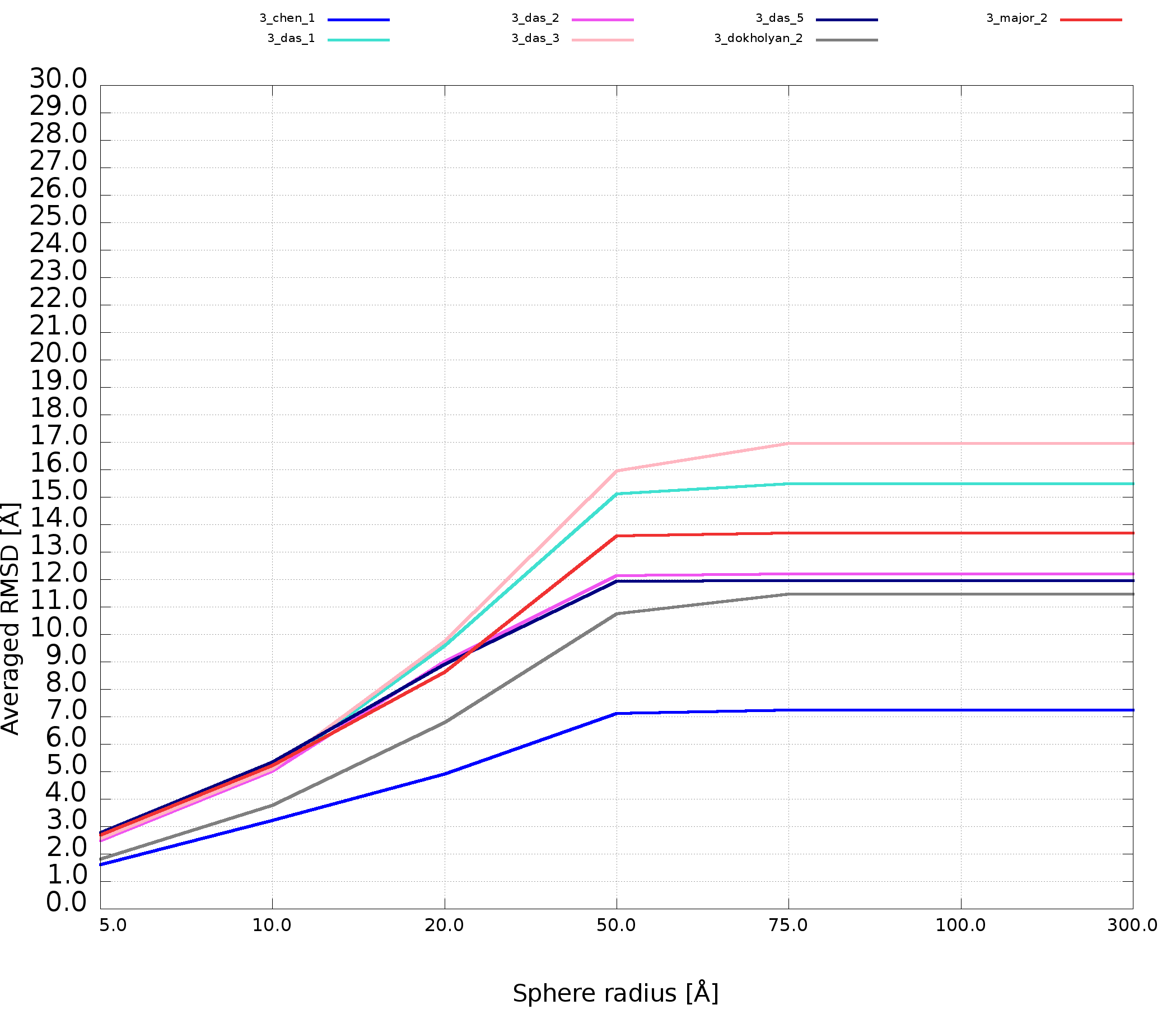Fig. 4. Averaged plot; X-axis represents the sphere radius, Y-axis represents averaged valuefor all spheres with fixed radius (different colors correspond to different models).

#### Cutoff plot

An example of a Cutoff plot is presented in Fig. 5. Cutoff plot shows how accurate is the prediction of a particular model from a local point of view or, in other words, which part of the model structure is predicted correctly. The calculation is performed based on the fixed precision value (cutoff) for each sphere radius value, defined by the user in spheres radii vector. As a result, the user receives information about the atoms set predicted below cutoff (%). The value of cutoff can be changed interactively by the user.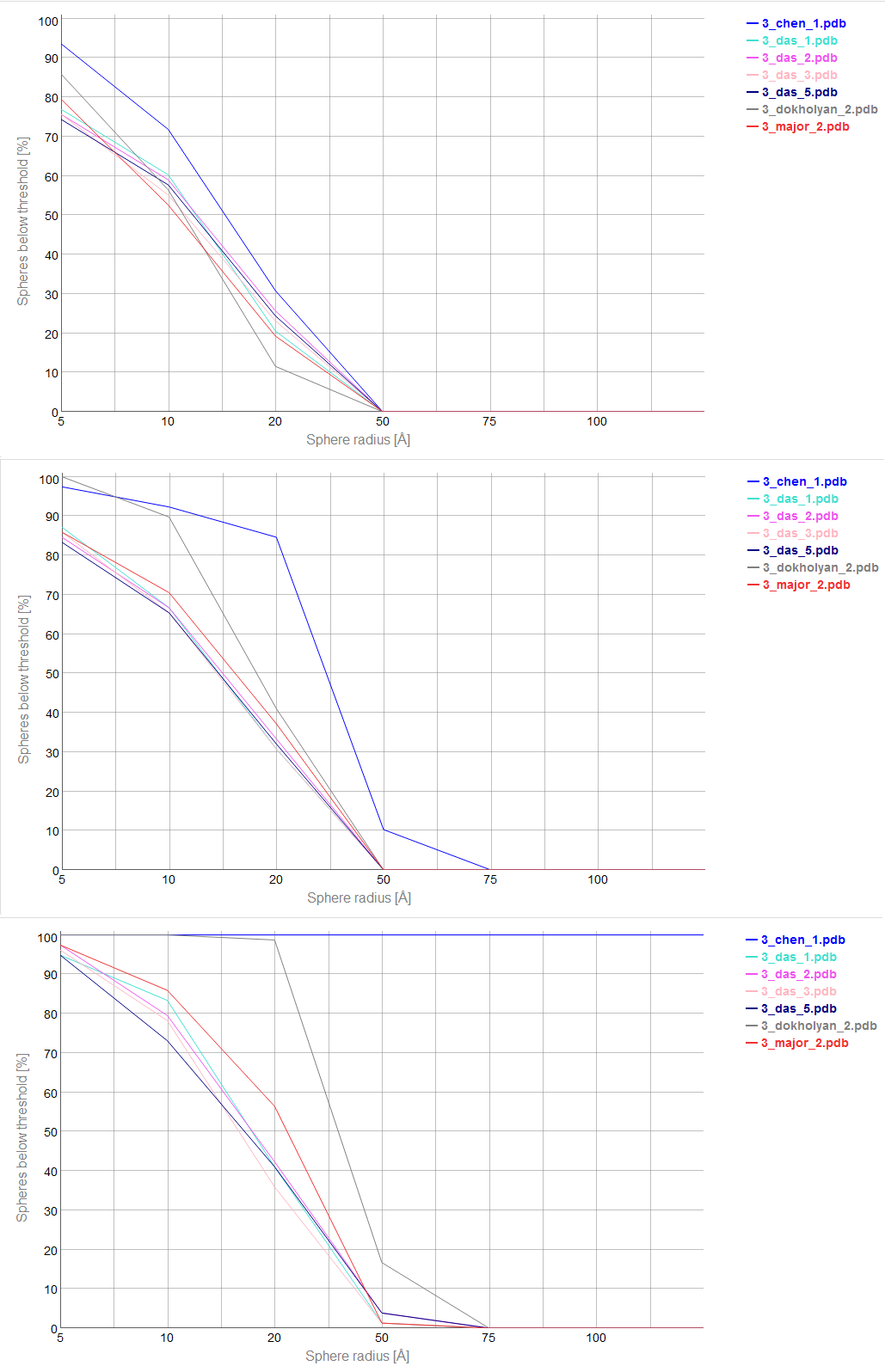Fig. 5. Cutoff plot presents a percentage of the number of atoms sets included in spheres with fixed radius for each considered model, which are below a selected precision value. One line corresponds to one model. X-axis corresponds to sphere value, Y axis corresponds to percentage value. On the picture above one can see plots for precision values (from top to the bottom: 4 Å, 7 Å and 10 Å).

#### Multi-model plot

An example of Multi-model plot is presented in Fig. 6. This is a multiple model line plot, where each line describes a single model analyzed. Each model is shown in a different color. Metric values between atoms from the reference structure and corresponding atoms from the model analyzed are calculated for atoms included in the sphere, where the center of the sphere is a selected atom (predefined by the user). Spheres are built for every nucleotide in the considered reference structure.

The Multi-model plot visualizes how accurate is the prediction of atoms located in the neighborhood of every nucleotide, taking into consideration the sphere radius (each line describes exactly one structural model - different colors correspond to different models). With a low radius (small local neighborhood – high precision of assessment), the local quality of models analyzed is very good for almost all nucleotide residues in the model, because accurate modeling of chemical structures of nucleotide residues is relatively easy. With the increasing value of radius one can easily identify the parts of models that exhibit different accuracies of prediction, from low values that indicate correct predictions, to important structural errors (for example different torsion angles – high values for local neighborhood) that should be widely and carefully analyzed. If the radius increases to match the molecule radius (i.e. at the very low level of precision of assessment), Value for the sphere that contains all nucleotides levels off to the same value, equal to global for a particular model (Fig. 6E).

The main feature of the Multi-model plot is Y-axis scaling of all predicted models to the potentially worst model, because its values are the highest. Visualization of two prediction models that differ significantly (one model – very good quality of prediction, second model – significant structural errors) on the Multi-model plot can be confusing because the value for the worse model may dominate the visualization of errors for the model with a better accuracy. Hence, the user can analyze each model separately.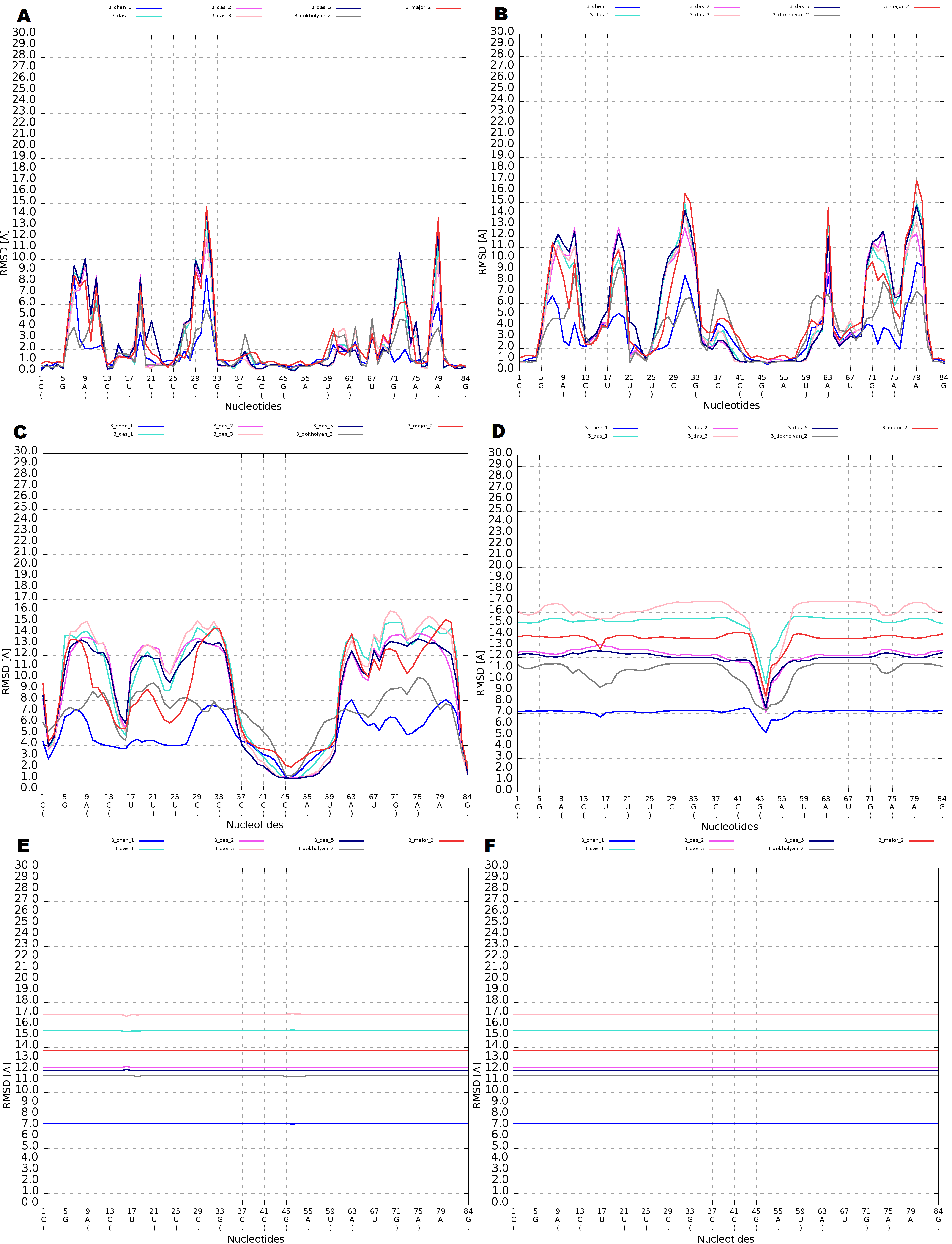Fig. 6. Multi-model plot for Challenge_3 of RNApuzzless contest; each plot (A-E) represents results for a different sphere radius (5, 10, 20, 50, 75 and 100 Å). X-axis represents the order of nucleotides in the sequence, Y- axis represents the value of RMSD.

#### Interaction with plots

Multimodel and cutoff plot can be adjusted be the user by changing the value of the sphere radius using slider above the plot or by setting particular value in the window Fig. 7.

Range selector bellow plot area can be used to filter nucleotides for which values are shown.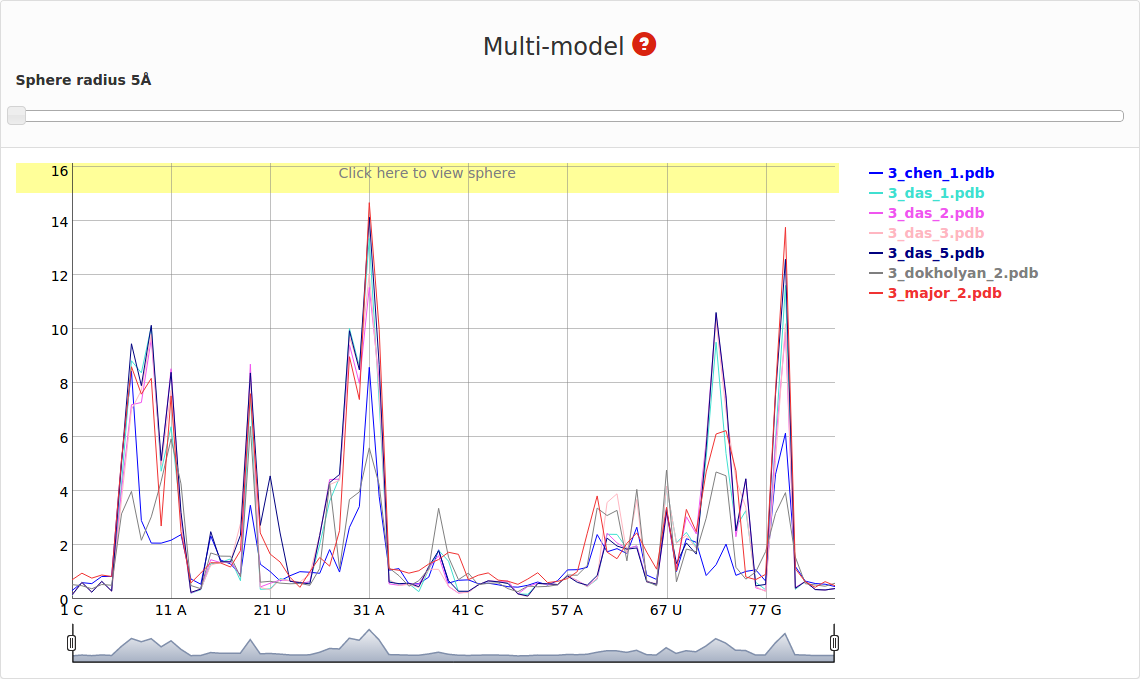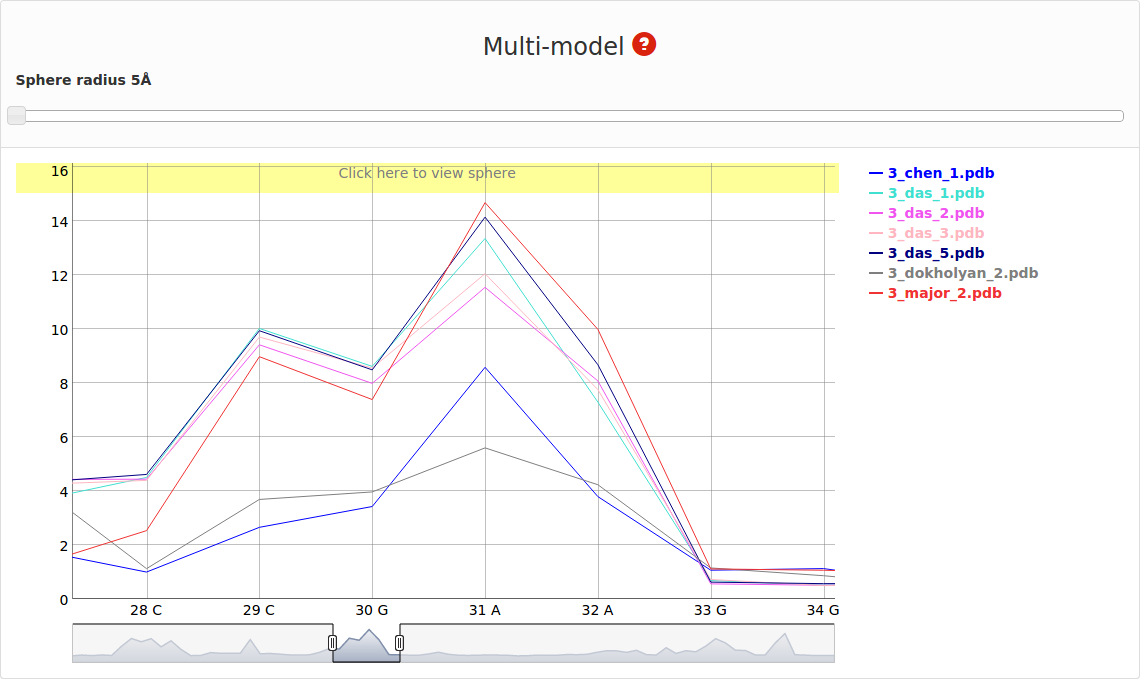Fig. 7. Multimodel plot (interactive)

On the right side one can see the legend that corresponds to each model Fig. 8.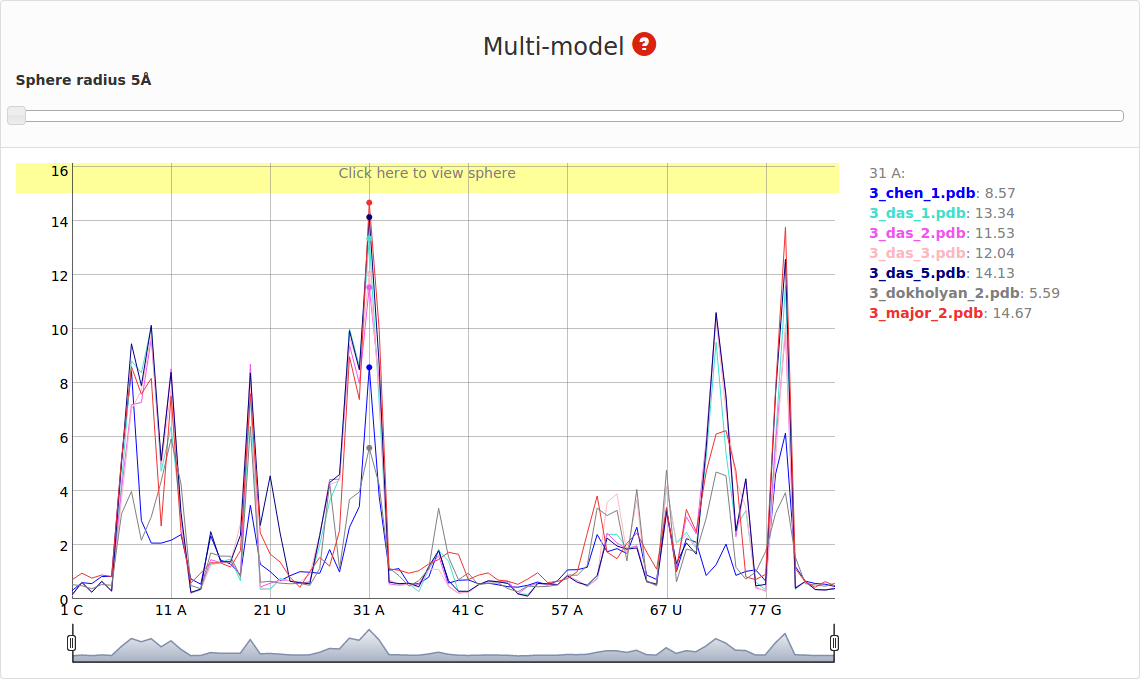Fig. 8. Multimodel plot (interactive) – dots correspond to the analyzed nucleotide.

If mouse pointer will be placed on the graph, the corresponding values as well as position will be presented on the right side of the model name. If user uses left mouse button having mouse pointer on the yellow bar (top of the plot), the visualization of the spheres corresponding to analyzed models built on particular nucleotide will be presented using Jmol software (Fig. 9). Left panel consists of list of models as well as visualization mode. Playing with checkboxes one can set what models will be visualized (by default all models will be shown (Fig. 9. left). If all checkboxes are unselected, only reference structure will be presented (Fig. 9., right)). Visualization mode give a possibility to view presented structure as cartoon or full atoms molecule. User should realize that presented structure corresponds to the set of atoms that are situated inside particular sphere. Only if the radius of sphere is larger enough to cover whole structure, the full model will be presented.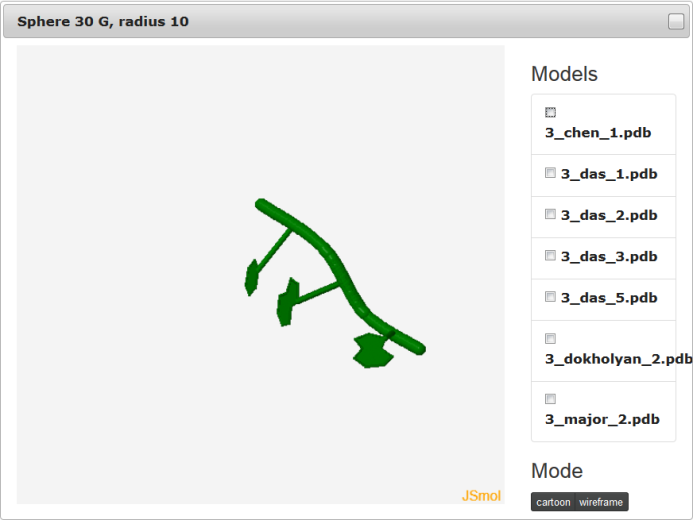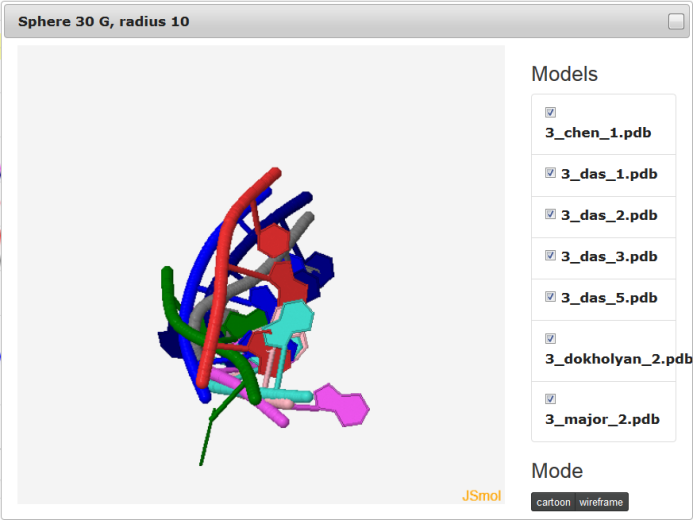Fig. 9. Visualization of spheres with Jmol.

The feature to visualize analyzed structures using Jmol is available only for Multimodel interactive plot.

#### Ad c) 2D and 3D graph section

That section presents 2D map plot and 3D graph for each analyzed model. Each row corresponds to one model. The data are presented in the following order: model’s name, 2D map, 3D plot.

#### 2D map plot

An example of a 2D map plot is presented in Fig. 10. This plot visualizes exactly one model at a time. The values are represented with a colored scale - values correspond to colors from blue (high prediction quality) to red (low prediction quality). The X-axis represents residue numbers (in sequential order), and the Y-axis represents the radius values from the spheres radii vector defined by the user. This plot shows where the prediction is inaccurate and allows the user to check if prediction errors are similar for different structures.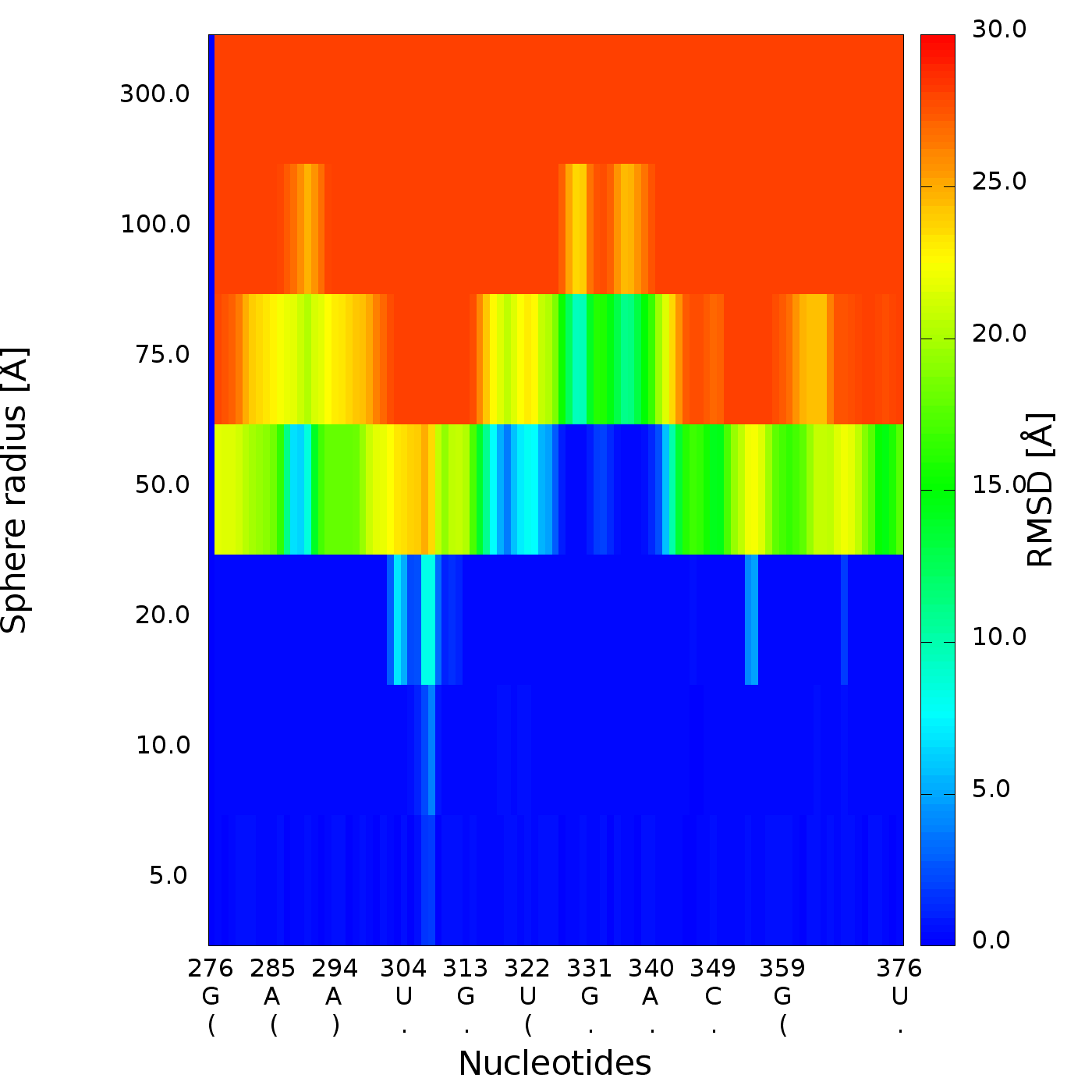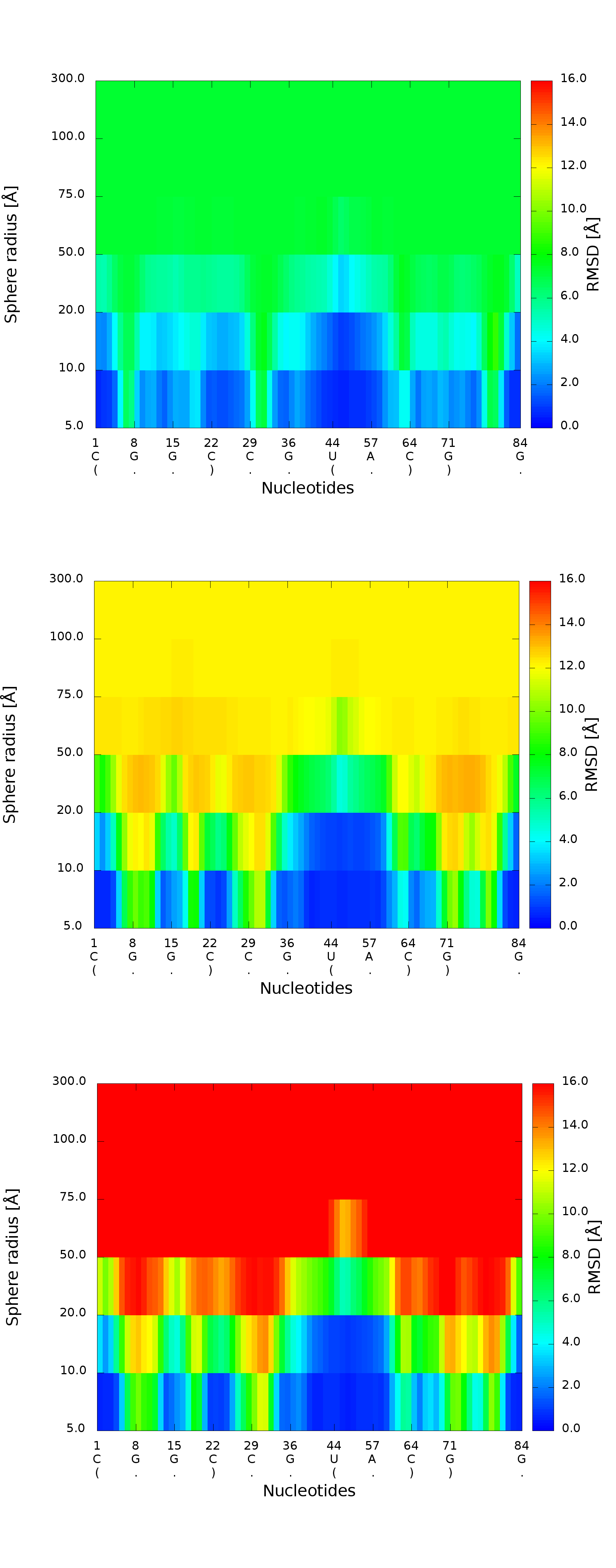Fig. 10. 2D map plot - each map corresponds to one of the analyzed models; X-axis represents the order of nucleotides in the sequence, Y-axis represents the sphere radius, color of the cell represents the RMSD value, following the scale presented at the bottom (blue – low RMSD, red – high RMSD).

#### 3D plot

An example of a 3D plot is presented in Figure 4. The X-axis represents nucleotide numbers in sequential order, the Z-axis represents the radius values from the spheres radii vector defined by the user, and the Y-axis represents RMSD. The value of RMSD is also represented with the colored scale. Both plots (2D map plot and 3D plot) describe the accuracy of fragments of a prediction model around certain nucleotides.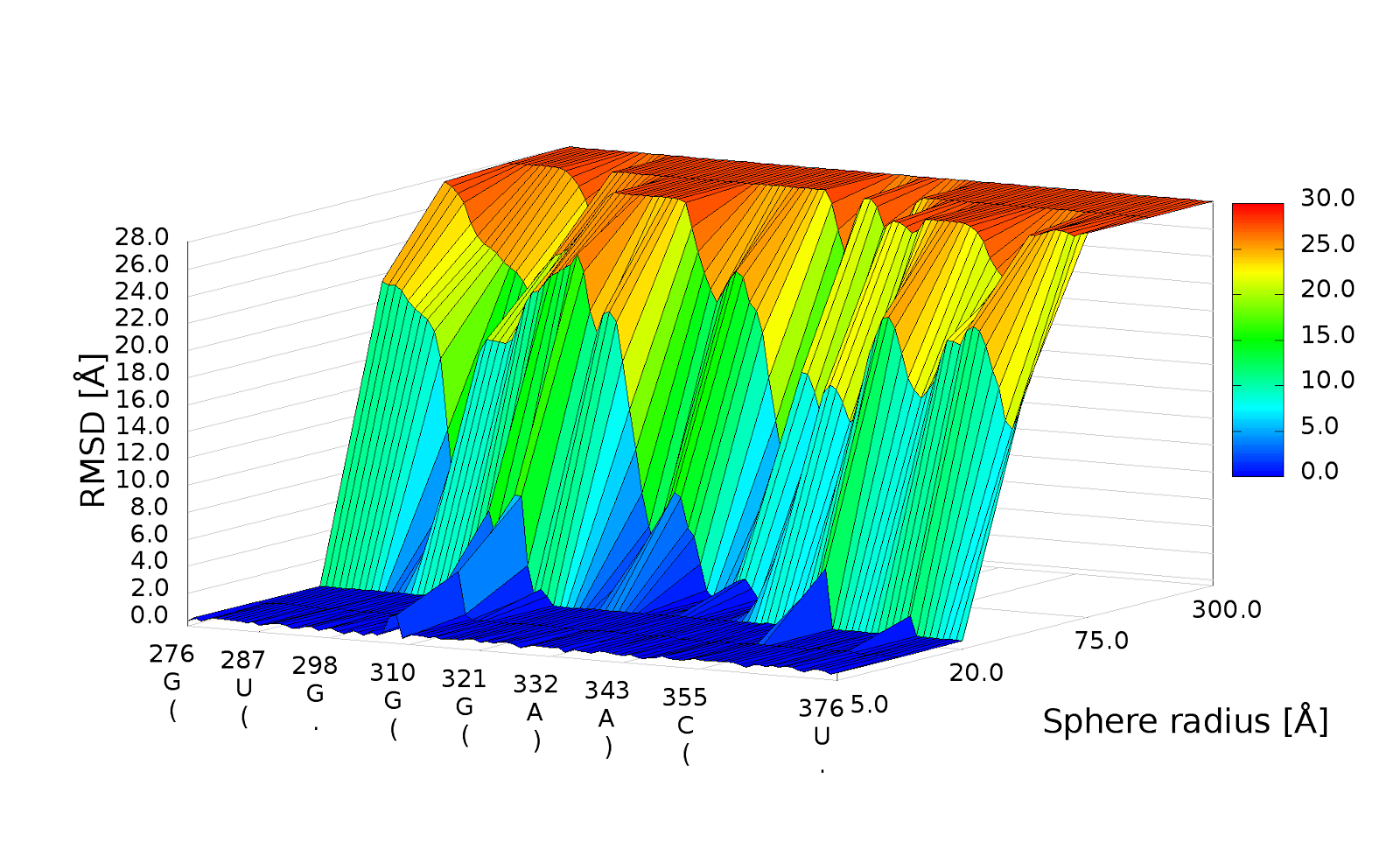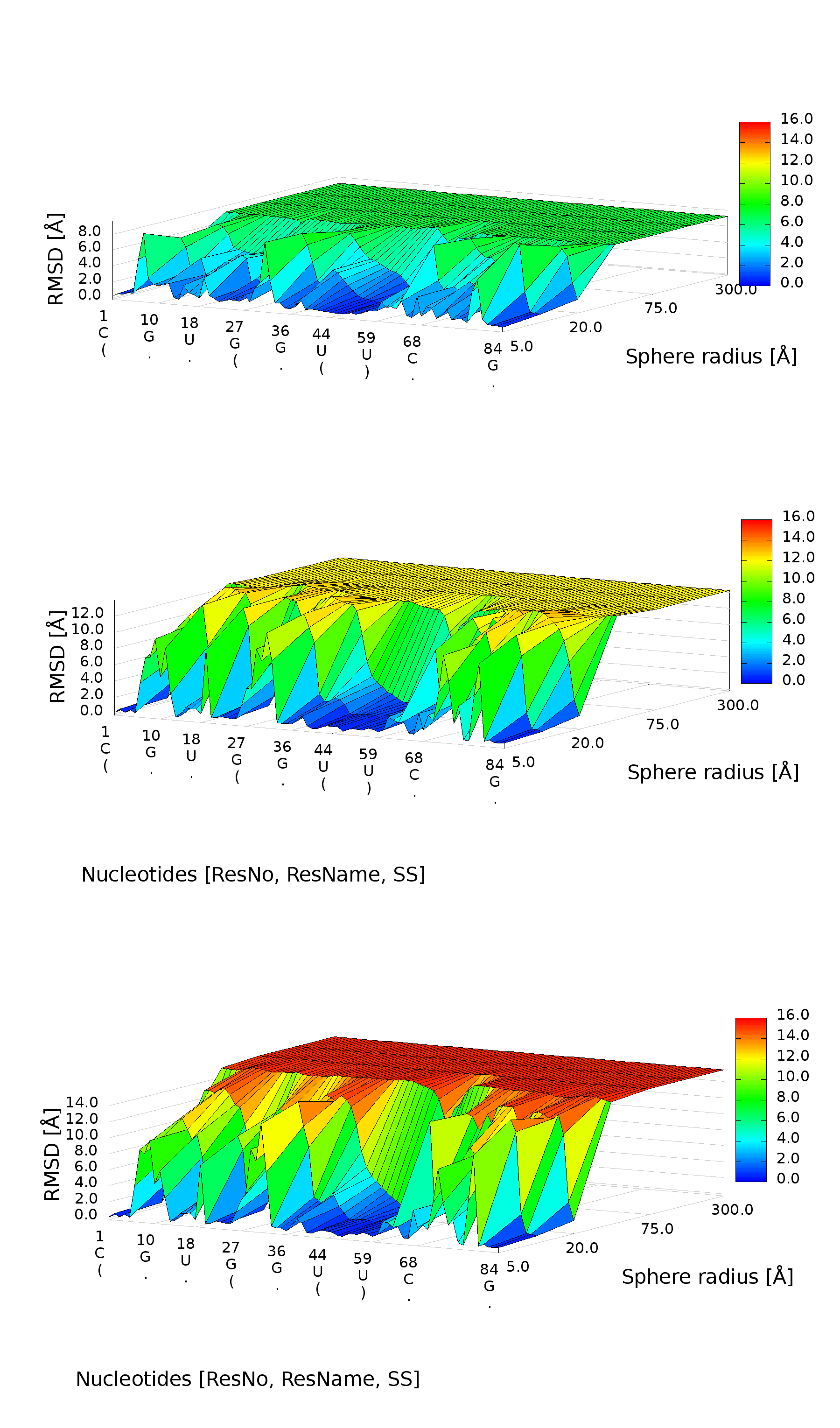Fig. 11. 3D plot – analysis of three models; X-axis represents the order of nucleotides, Y-axis represents sphere radius, Z-axis represents RMSD.

## Interpretation of the results

### Problem 1/Challenge case 1

The crystal structure of the regulatory element from human thymidylate synthase mRNA (29) revealed a dimer of identical sequences, with two asymmetrical internal loops. We analyzed all fourteen models submitted for this reference structure with the RNAssess, using the Multi-model plot.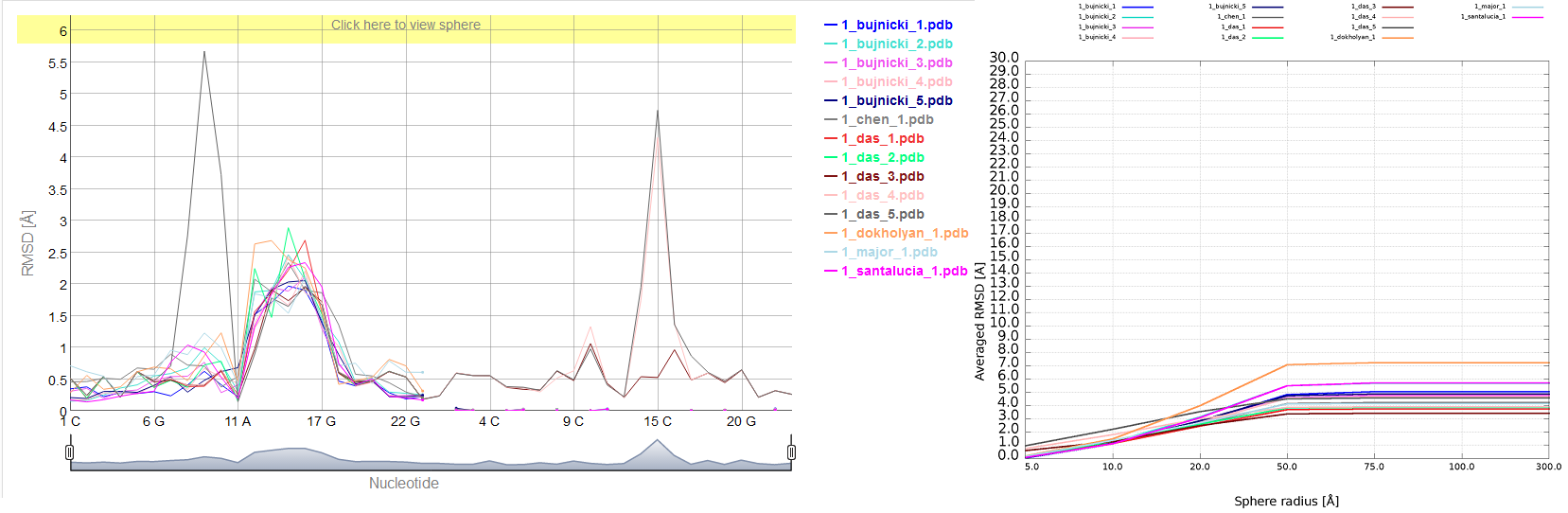Problem 1 – Multi-model plot (left) – prediction errors for all models are indicated in two regions. RMSD averaged plot (right)– for low sphere radius Bujnicki_model_1 is the best; different colours correspond to different models.

The analysis revealed that while all the models exhibit an approximately correct global structure, they show local errors in two positions (around residue 15 and 38), which is particularly evident in the plots for the sphere radius equal to 5 Å. For Das_model_4 and Das_model_5 models wrong local prediction is clearly visible in comparison with other models.

Looking at RMSD averaged plot, we can observe that local prediction in local neighborhood (up to 10 Å) is the best for Bujnicki_model_1 and Bujnicki_model_3 models. Analysis of Cutoff plot illustrates that local structural inconsistencies identified in Das_model_3 are compensated by an impressive quality of predictions for other regions, the percentage of spheres below cutoff threshold growing rapidly with the increasing value of radius.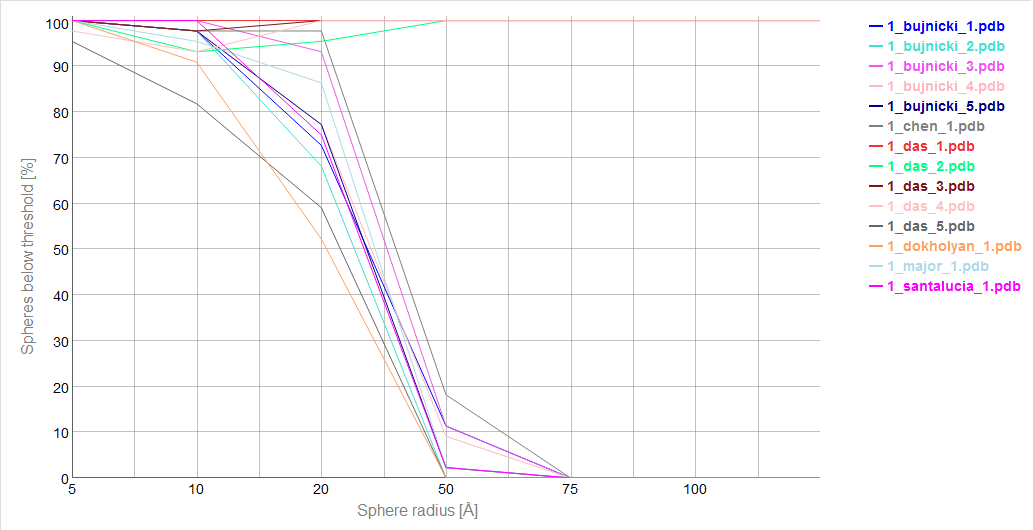Cutoff plot shows quite impressive local accuracy of 1_das_3 (precision 4 Å); different colours correspond to different models.

3D plots indicate structural differences between both predictions.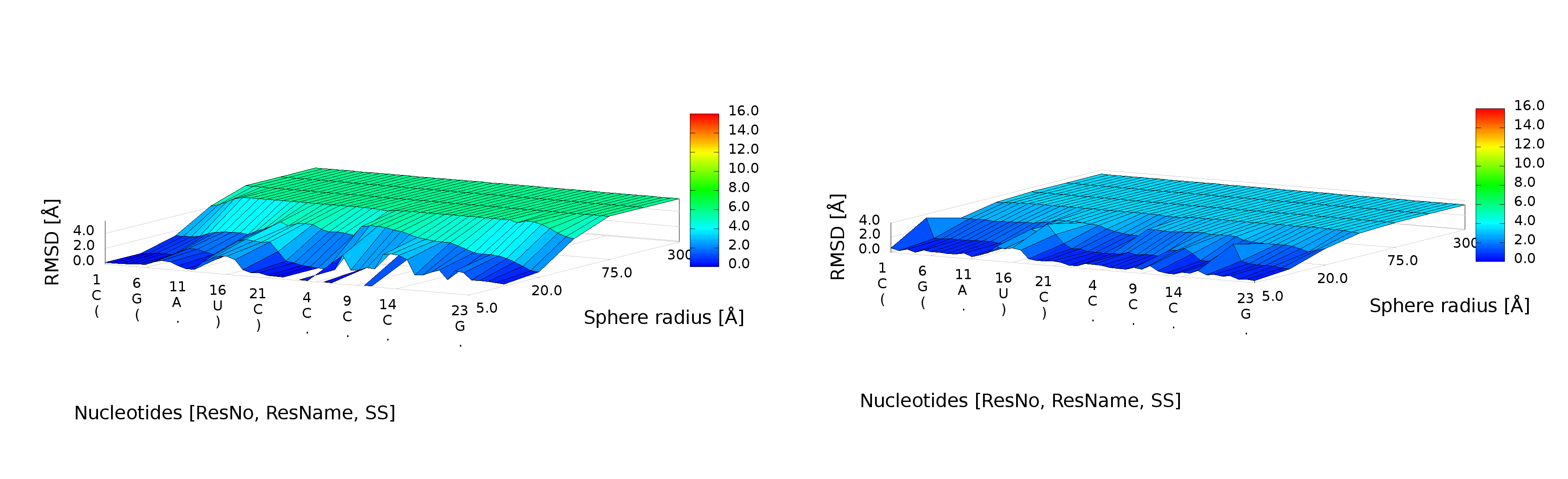3D plot of Santalucia_model_1 (left) and Das_model_3 (right).

Das_model_3 is potentially the best predicted model. Santalucia_model_1 has some missing atoms in regions that were generally difficult to model.

A detailed analysis of submitted models with RNAssess shows that this program can vividly point out to local conformations that were predicted much more accurately in globally less accurate models than their counterparts in globally more accurate models. This can be illustrated by e.g. comparison of Das_model_4 and Dokholyan_model_1 models.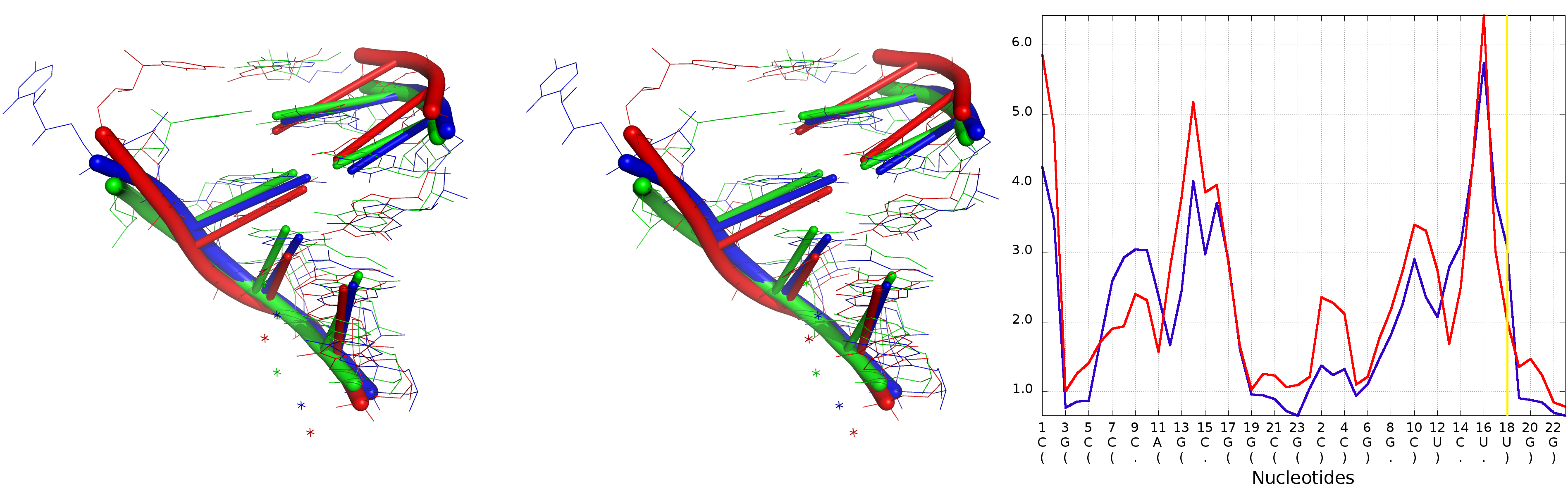Superposition of Das_model_4 and Dokholyan_model_1 (left) with the reference structure, Multi-model plot (right) corresponds to discussed regions (green colour – reference structure, red colour – globally less accurate model (Dokholyan_model_1), blue colour – globally more accurate model (Das_model_4).

The difference of RMSD for local neighborhood around nucleotide No 18 between analyzed models is over 1 Å (for the structural motif from Dokholyan_model_1 lower RMSD was calculated), but globally the RMSD value for the Das_model_4 is around 3 Å lower than the Dokholyan_model_1.

### Problem 2 / Challenge case 2

The reference molecule submitted for this challenge includes eight chains forming a square-like structure. The conformation of four shorter chains was provided, along with secondary structure, and the most important task was to model the remaining four chains, in particular loop regions. We analyzed 12 models submitted is response to this challenge. The input RNA molecule is larger than RNA molecule from Problem 1, so the total number of local structural errors is also much larger, but the impact of these errors on the global accuracy of the models is smaller, most likely owing to the constrains of the starting structure.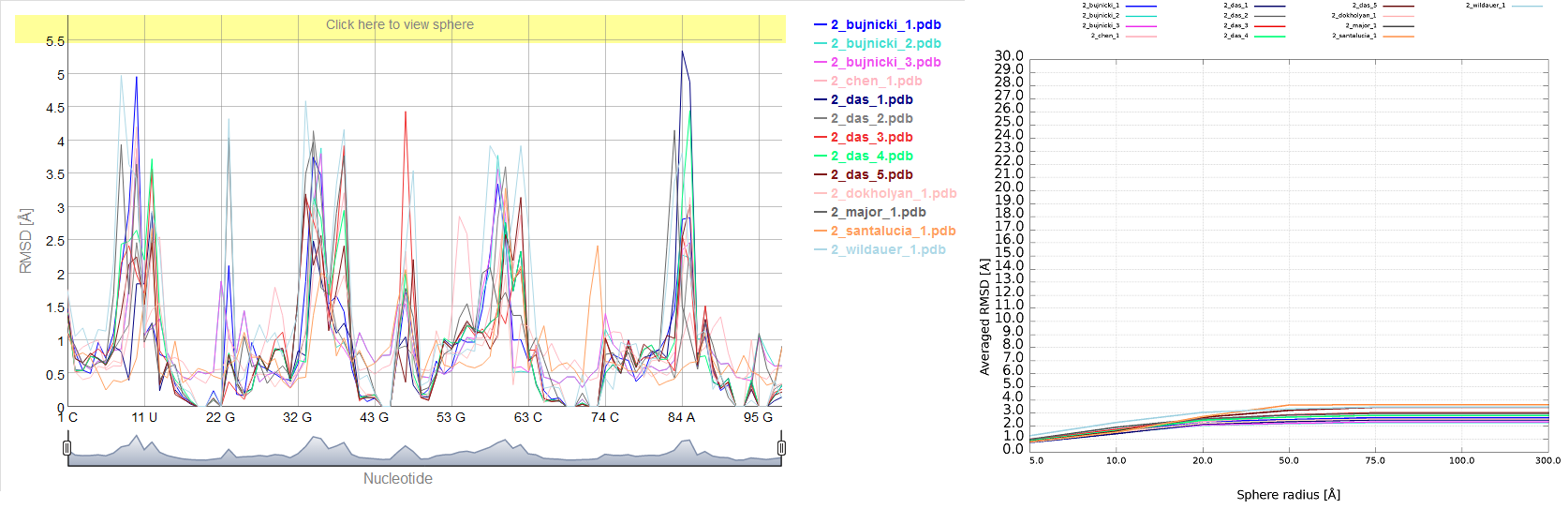Problem 2 – Multiple 1D plot (left) – prediction errors for all groups are indicated in several parts of the structure. RMSD averaged plot (right)– the Dokholyan_model_1 is the best for low sphere radius; different colours correspond to different models.

The average of local RMSD computed for low values of sphere radius gives advantage to Dokholyan_model_1, but with increasing radius of the sphere the Bujnicki_model_2 and Bujnicki_model_3 prove to be the best. Looking at Cutoff plot we can see that Das_model_1 is however the best one from the local perspective. 3D plots indicate structural differences between all predictions. On the other hand, in Bujnicki_model_3 and Bujnicki_model_2 models RNAssess points out local errors in several positions, which are however compensated on the global level by very accurate predictions in other regions.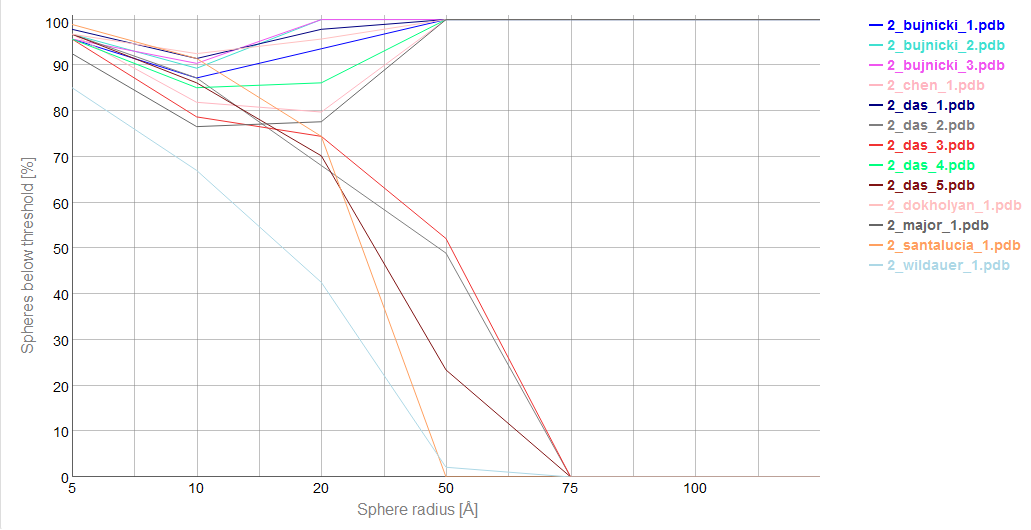Cutoff plot shows quite impressive local prediction of 2_das_1 (precision 3 Å); different colours correspond to different models.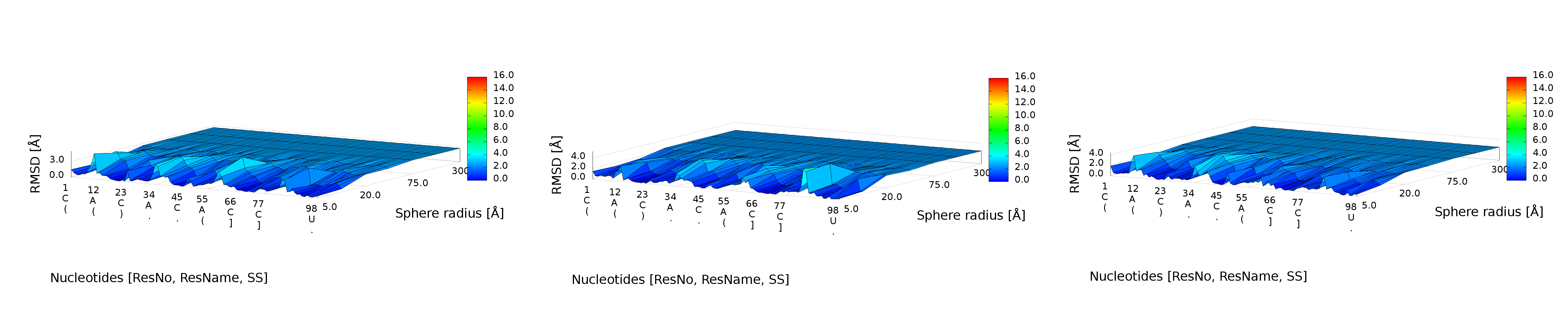3D plots of Bujnicki_model_1 (left), Das_model_1 (center) and Dokholyan_model_1 (right).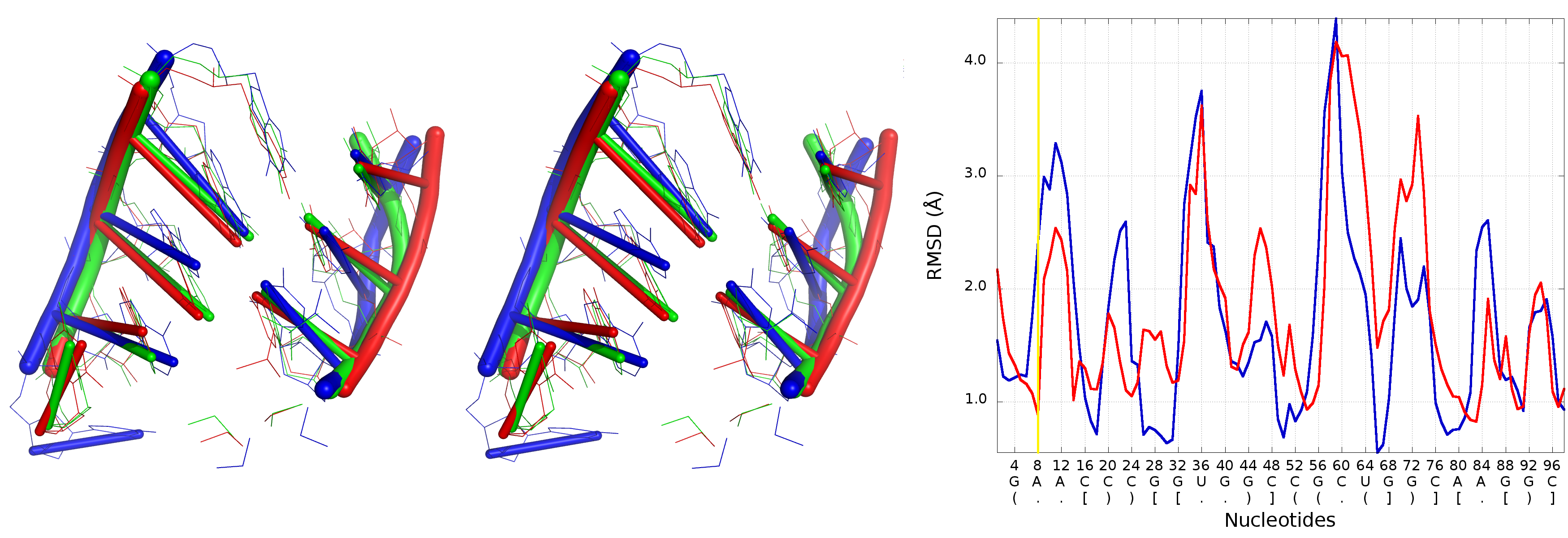Superposition of Bujnicki_model_2 and Santalucia_model_1 (left) with reference structure, Multi-model plot (right) corresponds to discussed regions (green colour – reference structure, red colour – globally less accurate model (Santalucia_model_1), blue colour – globally more accurate model (Bujnicki_model_2).

Further analysis illustrates that Bujnicki_model_1 in the local neighborhood located around nucleotide No 7 is actually worse than the globally less accurate Santalucia_model_1. The local RMSD between analyzed models is around 3 Å to the advantage of Santalucia_model_1, but globally the RMSD calculated for Bujnicki_model_1 is better by 1 Å than Santalucia_model_1.

### Problem 3 / Challenge case 3

The glycine riboswitch structure is a relatively large RNA molecule and its prediction presented considerable challenge, compared to the other considered problems. Twelve models have been submitted for this target. Predicted models are relatively far from the reference structure and the structural differences between the quality of submitted models are quite large.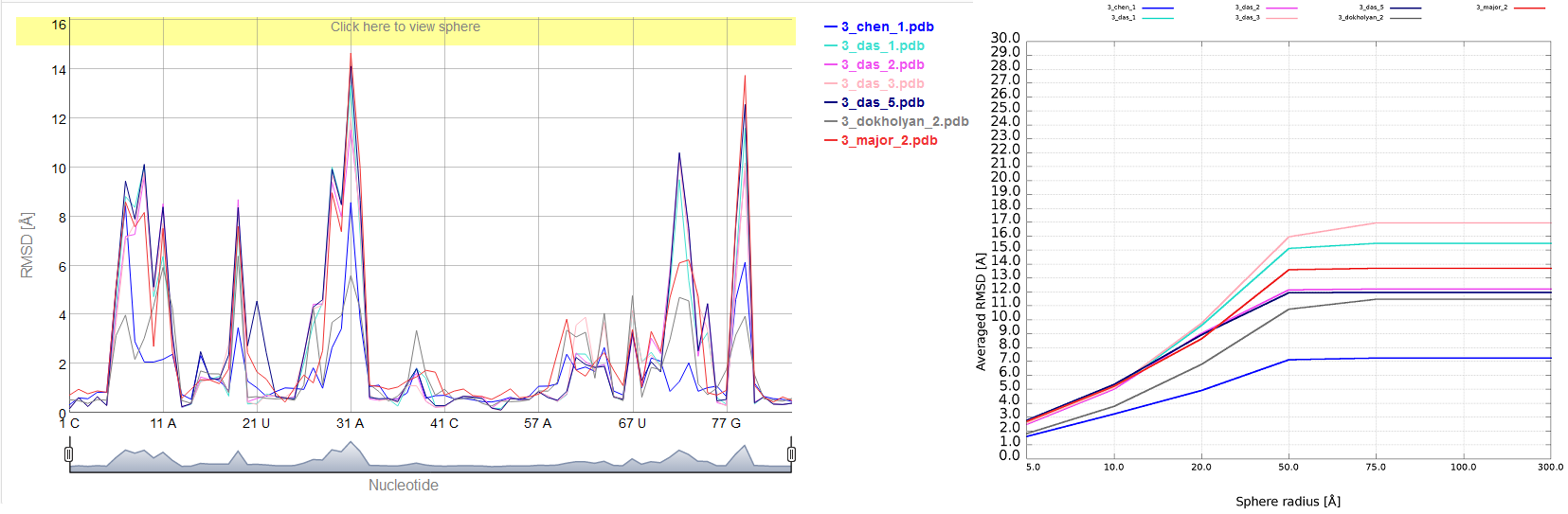Problem_3 – Multi-model plot (left) – prediction errors for all groups are indicated in several regions. RMSD averaged plot (right) – for low sphere radius values Chen_model_1 is the best; different colours correspond to different models.

The analysis of the local neighborhood shows that there were submitted two models with similar prediction quality identified for spheres with radius up to 10 Å (Chen_model_1 and Dokholyan_model_1).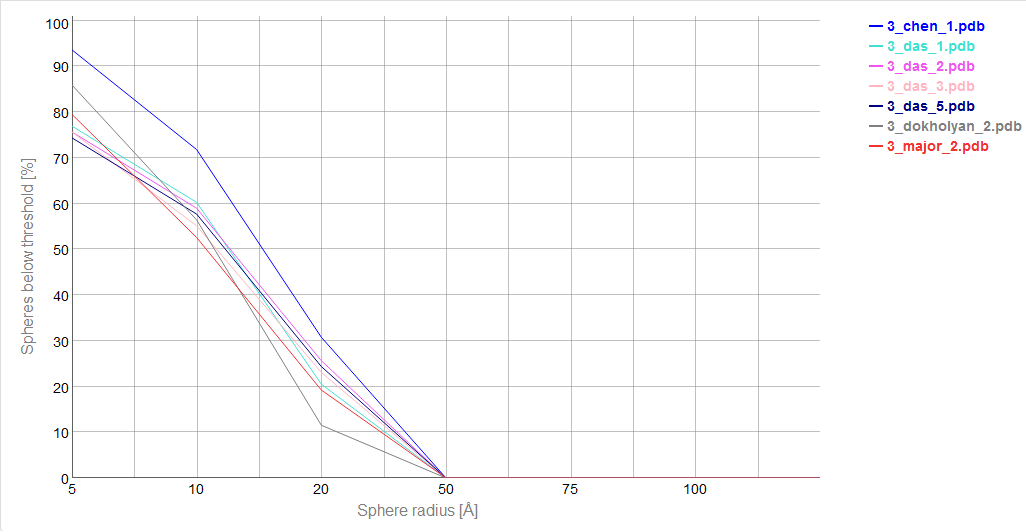Cutoff plot ilustrates outstanding local prediction of 3_chen_1 (precision 4 Å); different colours correspond to different models.

For a larger neighborhood (lower accuracy level) Chen_model_1 considerably surpassed all other models.

3D plots show that Dokholyan_model_1 is quite worse than Chen_model_1.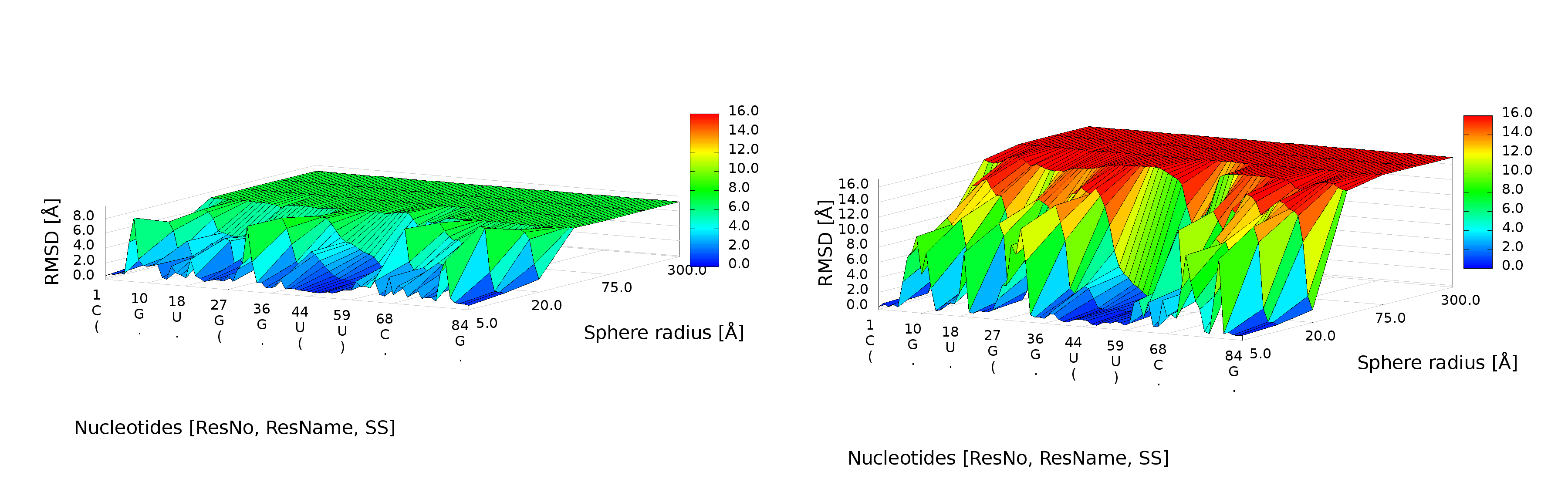3D plot of Chen_model_1 (left) and Dokholyan_model_1 (right).

Detailed analysis illustrates that the RMSD computed for Bujnicki_model_1 in the local neighborhood around nucleotide No 42 is higher than for corresponding structural fragments identified in Das_model_3. The difference between the superimposed corresponding structural fragments is over 1 Å, but globally the RMSD for Bujnicki_model_1 is lower by 4 Å.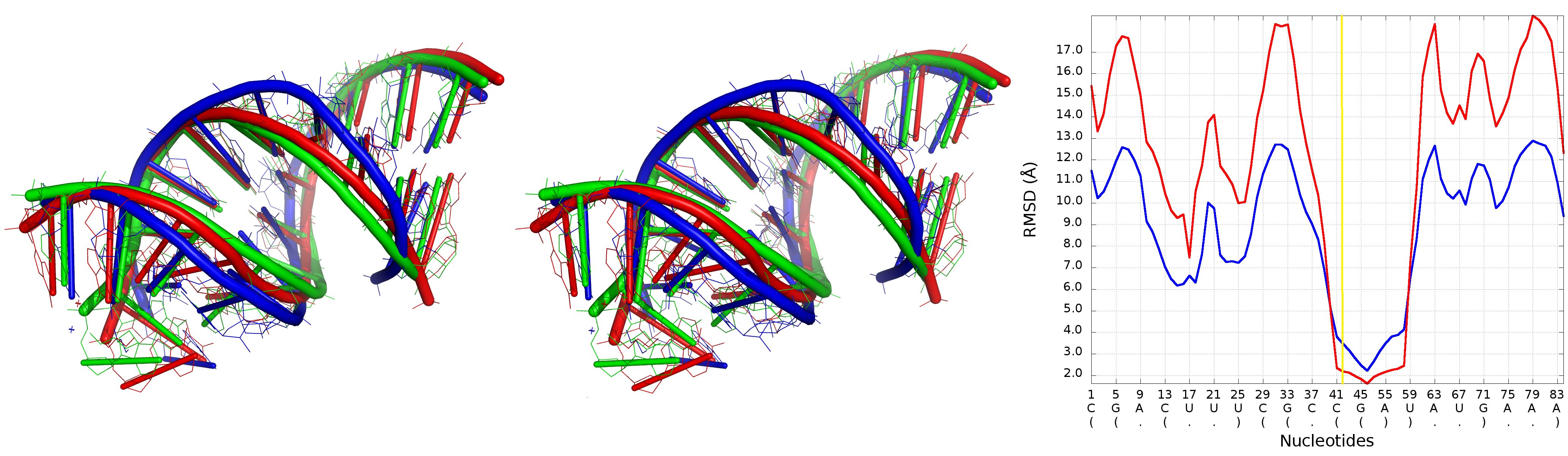Superposition of Bujnicki_model_1 and Das_model_3 (left) with reference structure, Multi-model plot (right) corresponds to discussed regions (green colour – reference structure, red colour - globally less accurate model (Das_model_3), blue colour – globally more accurate model (Bujnicki_model_1).